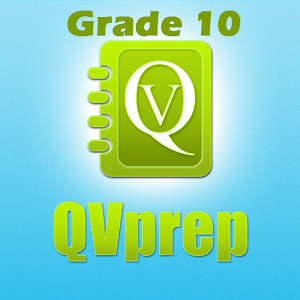# 10th grade math english ten 10 - Lumos Educational App StoreNA1
Price -NA
\$A

#### DESCRIPTION:

If you practice hard, you can excel !! QVprep for grade 10 provides you hundreds of math and english problems with detailed answer explanations so that you can create a strong learning foundation. QVprep 10th grade Quantitative Maths Verbal English Ability Practice Test prep for tenth grade 10 math vocab quiz common core standard QVprep 10 for tenth grade helps students to practice, learn and improve their knowledge in maths and english. The app has hundreds of multiple choice questions with answers in 20 practice tests on quantitative (maths) and verbal (english) ability. This app is idea

#### OVERVIEW:

10th grade math english ten 10 is a free educational mobile app By PJP Consulting LLC.It helps students in grades HS practice the following standards HSN.RN.A.1,HSN.RN.A.2,HSN.RN.B.3.

This page not only allows students and teachers download 10th grade math english ten 10 but also find engaging Sample Questions, Videos, Pins, Worksheets, Books related to the following topics.

1. HSN.RN.A.1 : Explain how the definition of the meaning of rational exponents follows from extending the properties of integer exponents to those values, allowing for a notation for radicals in terms of rational exponents. For example, we define 51/3 to be the cube root of 5 because we want (51/3)3 = 5(1/3)3 to hold, so (51/3)3 must equal 5.

2. HSN.RN.A.2 : Rewrite expressions involving radicals and rational exponents using the properties of exponents..

3. HSN.RN.B.3 : Explain why the sum or product of two rational numbers is rational; that the sum of a rational number and an irrational number is irrational; and that the product of a nonzero rational number and an irrational number is irrational.

HS

#### STANDARDS:

HSN.RN.A.1
HSN.RN.A.2
HSN.RN.B.3

Developer: PJP Consulting LLC

Developer URL: http://www.QVprep.com

Software Version: 1

Category: Education

### RELATED APPS